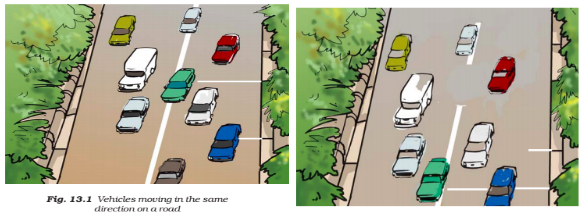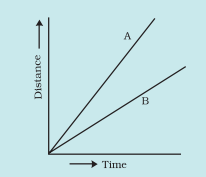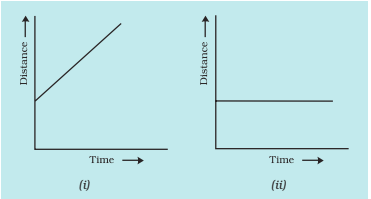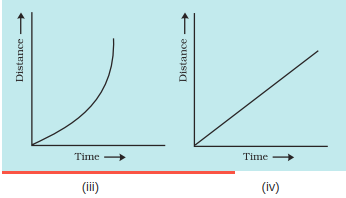# NCERT Solutions for Class 7 Science Chapter 13 Motion and Time

NCERT solutions for class 7 science chapter 13 Motion and Time: After completing a chapter it is necessary to practice the questions given in the book. Solutions of NCERT class 7 science chapter 13 motion and time helps students in solving the homework questions. Earlier you have learnt about different types of motions. You learnt the motion along a straight line, circular path and periodic motion. The first question explained in the CBSE NCERT solutions for class 7 science chapter 13 motion and time is based on these classifications. You know that some vehicles move faster than others. If you know the distance covered by two buses in one hour, it is easy to tell which one is faster. The distance covered by an object per unit time as the speed. All you will study in this chapter is the speed and time measurements. NCERT solutions for class 7 science chapter 13 motion and time is helpful in scoring good marks in the examination. Solutions of NCERT is an essential tool for the preparation of the exam.

Here are the important topics of NCERT class 7 science chapter 13 motion and time

13.1 Slow or Fast

13.2 Speed

13.3 Measurement of Time

Units of Time and Speed

13.4 Measuring Speed

13.5 Distance-time Graph

If you have any problem in answering the exercise questions or you are not getting the correct answers then don't worry, NCERT solutions for class 7 science chapter 13 motion and time is there for you to help.

## Solutions of NCERT for class 7 chapter 13 motion and time exercise:

(i) Motion of your hands while running.
(ii) Motion of a horse pulling a cart on a straight road.
(iii) Motion of a child in a merry-go-round.
(iv) Motion of a child on a see-saw.
(v) Motion of the hammer of an electric bell.
(vi) Motion of a train on a straight bridge.

(i) Oscillatory motion
While running, our hands move in back and forth direction and repeat its movement after some time. Hence it is an oscillatory motion.

(ii) Motion along the straight line-
A horse is pulling a cart on a straight road. Therefore it follows the motion along a straight line.

(iii) Circular motion-
Since the merry-go-round moves in a circular motion

(iv) Oscillatory motion-
The see-saw moves up and down continuously. It oscillates up-down.

(v) Oscillatory motion
(vi) Motion along a straight line-
The train is moving on a straight bridge. Therefore it follows the motion along the straight line.

(i) The basic unit of time is second.
(ii) Every object moves with a constant speed.
(iii) Distances between two cities are measured in kilometres.
(iv) The time period of a given pendulum is constant.
(v) The speed of a train is expressed in m/h.

The option (ii), (iv) and (v) are not correct.

(ii) The object can move with variable or constant speed.

(iv) The time period of a pendulum depends on the length of the thread. So, it is constant for a particular length.

(v) Speed of train is measured either in km/h or m/s not in m/h

Given that,
A simple pendulum takes 32 s to complete 20 oscillations. It means,
No. of oscillation = 20, and
Total time is taken = 32 s
According to question,
Time period = (T)

Given that,
Distance between the two stations = 240 km
and, time taken = 4 hour

Therefore, Speed = Distance / Time
= 240/4
= 60 km/h

We have,
The initial reading of odometer = 57321 km
The final reading of odometer = 57336 km

So, distance covered by the Car = Final reading - Initial reading = 15 km

The total time taken by the car to travel 15 km = 8:50 AM - 8:30 AM = 20 min

According to question,
Speed of the car  = Distance / time taken = 15/20 = 0.75 km/min

Again, 60 minute = 1 hr
so, 1 min = 1/60 hr

Speed in terms of km/hr =

Given that,
time taken by Salma to reach her school from house = 15 min =
and, speed of the bicycle = 2 m/s

It is known that,
Distance covered = Speed  time taken

= 1.8 km

(i) A car moving with a constant speed.
(ii) A car parked on a side road.

(i) A car is moving with a constant speed covers equal distance in equal interval of time. Therefore,(ii) Distance-time graph of a car parked on a roadside is such that an increase in time, there is no change in distance.## 8. Which of the following relations is correct?

The option (ii) is the correct answer.
Speed = distance /time , this relation holds correctly

## 9. The basic unit of speed is:

(i) km/min     (ii) m/min      (iii) km/h     (iv) m/s

The option (iv) is the correct answer.
The SI unit of speed is m/s (meter per second)

(i) 100 km     (ii) 25 km    (iii) 15 km     (iv) 10 km

The correct option is (ii)

1st case,
Speed = 40 km/hr
and time taken = 15 min
So, distance =

Case 2nd,
Speed = 60 km/h
Time taken = 15 min = 0.25 h
So, Distance travelled =

So, total distance = 15 + 10 = 25 kmGiven, Scale:

The blue car is the fastest.
Distance travelled by the blue car in the picture=

Therefore, the actual distance travelled by blue car =

Time taken =

Speed of the blue car =

Therefore, Speed of the blue car isIt is known that,
(speed = distance/time)
it means greater the speed, more distance is covered by the car in a given interval of time.
From the graph, we can see that The distance covered by the vehicle A is more than the vehicle B. Hence A is faster than B.

## 13. Which of the following distance-time graphs shows a truck moving with a speed which is not constant?The correct option is (iii)

• In  graphs, the constant speed is shown by a straight line.
• A straight line parallels to the time axis, it indicates that the body is at rest position.
• A curved line on  graphs means the speed is not constant.

## Chapterwise NCERT solutions of class 7 science

 Chapter No. Chapter Name Chapter 1 NCERT solutions for class 7 science chapter 1 Nutrition in Plants Chapter 2 Solutions of NCERT class 7 science chapter 2 Nutrition in Animals Chapter 3 CBSE NCERT solutions for class 7 science chapter 3 Fibre to Fabric Chapter 4 NCERT solutions for class 7 science chapter 4 Heat Chapter 5 Solutions of NCERT class 7 science chapter 5 Acids Bases and Salts Chapter 6 CBSE NCERT solutions for class 7 science chapter 6 Physical and Chemical Changes Chapter 7 NCERT solutions for class 7 science chapter 7 Weather Climate and Adaptations of Animals to Climate Chapter 8 Solutions of NCERT class 7 science chapter 8 Winds storms and cyclones Chapter 9 CBSE NCERT solutions for class 7 science chapter 9 Soil Chapter 10 NCERT solutions for class 7 science chapter 10 Respiration in Organisms Chapter 11 Solutions of NCERT class 7 science chapter 11 Transportation in Animals and Plants Chapter 12 CBSE NCERT solutions for class 7 science chapter 12 Reproduction in Plants Chapter 13 NCERT solutions for class 7 science chapter 13 Motion and Time Chapter 14 Solutions of NCERT class 7 science chapter 14 Electric Current and its Effects Chapter 15 CBSE NCERT solutions for class 7 science chapter 15 Light Chapter 16 NCERT solutions for class 7 science chapter 16 Water A Precious Resource Chapter 17 Solutions of NCERT class 7 science chapter 17 Forests Our Lifeline Chapter 18 CBSE NCERT solutions for class 7 science chapter 18 Wastewater Story

## NCERT Solutions for Class 7 Subject Wise

 NCERT Solutions for Class 7 Maths NCERT Solutions for Class 7 Science

## Important formulas:

The numerical problems discussed in the CBSE NCERT solutions for class 7 science chapter 13 motion and time is based on the following formulas.

• Time period

• Questions based on the distance-time graph are also discussed in the NCERT solutions for class 7 science chapter 13 motion and time.